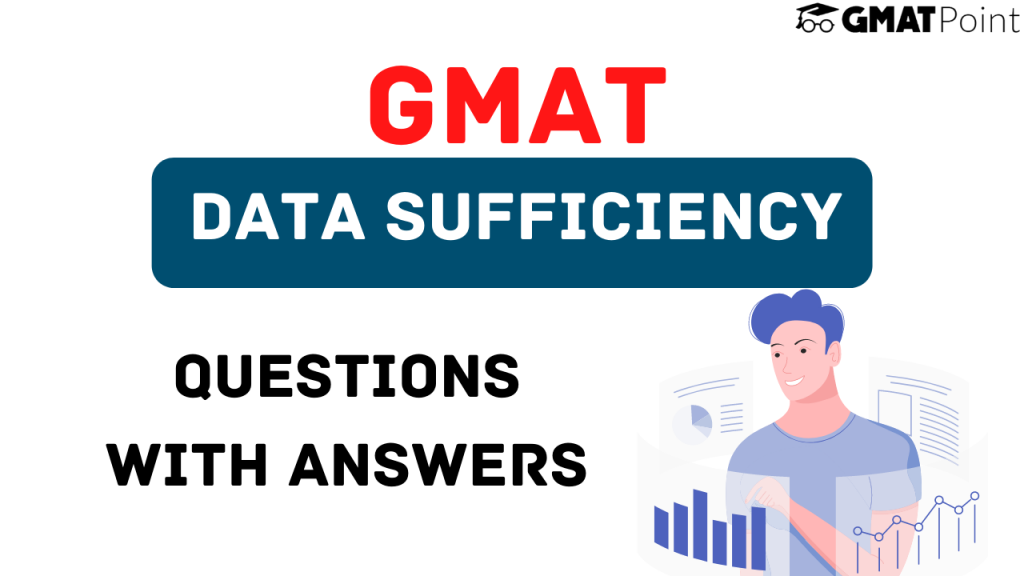# GMAT Data Sufficiency Questions With Answers# GMAT Data Sufficiency Questions With Answers

Data Sufficiency questions constitute a major chunk of the Quant section of the GMAT. Of the 31 questions that appear in this section, you can expect close to 50% of the questions from Data Sufficiency. In this article, we will be looking into 3 examples of GMAT Data Sufficiency Questions With Answers

In case you do not have your basics of Data Sufficiency cleared, I highly recommend going over our other blog on GMAT Data Sufficiency strategy.

## Examples – GMAT Data Sufficiency Questions With Answers

### Question 1:

Is xy < 6 ?

Statement 1: x< 2, y<3

Statement 2: x and y are non-negative

a) Statement (1) ALONE is sufficient, but Statement (2) alone is not sufficient
b) Statement (2) ALONE is sufficient, but Statement (1) alone is not sufficient
c) Both Statements (1) and (2) TOGETHER are sufficient, but NEITHER statement ALONE is sufficient.
d) EACH statement ALONE is sufficient
e) Statements (1) and (2) TOGETHER are NOT sufficient

### Approach:

Let us consider Statement 1. If x < 2 and y < 3, then xy < 6. But this is only possible if we do not consider the sign.

Imagine that one of them turns out to be negative, then irrespective of the other value, xy < 6.

However, if both turn out to be negative, then xy can take any value as large as possible.

Hence, Statement 1 is not sufficient.

Statement 2 is again not sufficient since x and y can take any value.

When we combine these statements, we know that x and y are either positive or zero. If any one or both are zero, xy < 6

When none of them is zero, they are still less than 6.

Hence, option C stating that both together are sufficient, however, none of them individually are sufficient is right.

### Question 2:

What is the radius of a circle C?

Statement 1: The ratio of the circumference and the diameter = 3.14

Statement 2: The ratio of the area and the circumference is 5.4 units.

a) Statement (1) ALONE is sufficient, but Statement (2) alone is not sufficient
b) Statement (2) ALONE is sufficient, but Statement (1) alone is not sufficient
c) Both Statements (1) and (2) TOGETHER are sufficient, but NEITHER statement ALONE is sufficient.
d) EACH statement ALONE is sufficient
e) Statements (1) and (2) TOGETHER are NOT sufficient

### Approach:

Statement 1: Circumference = 2 * pi * r

Diameter = 2 * r

Approach:Ratio = pi

The statement does not add any value to the information that is provided.

Statement 2: The ratio of the area and the circumference is 5.4 units.

pi * r * r / (2 * pi * r) = 5.4

r = 10.8 units.

Hence, Option B – Statement 1 alone is not sufficient, Statement 2 alone is sufficient is the correct choice.

### Question 3:

Is |x+y| > 5 ?
Statement 1: |2x| > 6
Statement 2: |y| > 2

a) Statement (1) ALONE is sufficient, but Statement (2) alone is not sufficient
b) Statement (2) ALONE is sufficient, but Statement (1) alone is not sufficient
c) Both Statements (1) and (2) TOGETHER are sufficient, but NEITHER statement ALONE is sufficient.
d) EACH statement ALONE is sufficient
e) Statements (1) and (2) TOGETHER are NOT sufficient

### Approach:

Statement 1: |2x| > 6

Hence, |x| > 3

Statement 2: |y| > 2

Now, for  |x+y| > 5, we must satisfy the following condition:

x + y < -5 or x + y > 5

Now, using both hints we know that |x| > 3 and |y| > 2.

If both x and y are negative, or both x and y are positive, we can definitely say that x + y > 5 or x + y < -5

Hence, |x + y| > 5.

But if one of them is positive and the other is negative, there can e several cases possible, if we can find out even a single case where |x + y| < 5, we can safely say that both the options together are not sufficient.

Suppose x = 6 and y = -4, then |x+y| = 2, which is less than 5, hence, option E is the correct choice.

### Note:

Though these examples provide a good sense of what type of GMAT Data Sufficieny questions you can expect, in no way do they represent the exhaustive list of concepts required for the Quantitative section of GMAT.

You can check out the Free GMAT Daily Targets on our platform.

Also, check out the Free GMAT Verbal Tests and Quant Tests.

If you are starting your GMAT preparation from scratch, do check out GMATPOINT.